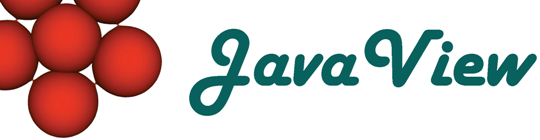Overview  Gallery  Introduction  Objects  Packages  Packages 3D  Basic Options  3D Options  Advanced

## 1. Introduction

Mathematica graphics objects cover a broad range of 2D and 3D shapes. A single command like JavaView[g] suffices to transfer these shapes to JavaView as interactive geometries. Behind the scenes JavaView analyzes the graphics object g, parses its internal representation, and, in some case, performs necessary cleanup computations.

The purpose of the following sections is to demonstrate that a variety of Mathematica graphics primitives are successfully parsed by JavaView, and that JavaView renders the shapes as expected by a Mathematica user and as defined by the rendering options included in the graphics objects. Each example below links to a separate page which compares the rendering of a Mathematica graphics in a JavaView and a JavaView-Lite applet with an image originally rendered by Mathematica.

Mathematica and JavaView deeply integrate via the J/Link connection, for example, changes on a geometry performed in JavaView may be returned to a Mathematica session. In order to focus on the parsing and display issues we constrain to the use of the command JavaView[] in this text.

 JavaView[g] Show a graphic object in an interactive JavaView display. JavaView[{g1,g2,..}] Show a list of graphics objects as scene in a display. JavaView[{g1,..}, Animatable->True] Show a list of graphics objects as smooth animation transforming into each other. WriteMgs[g, file] Save a graphics to a file with filename extension *.mgs. Such files can be shown in JavaView applets. WriteHtml[g, file] Create a web page which displays the graphics in a JavaView applet.
JavaView commands used in this section.

#### Remark on this Applet Version

The shown models are precomputed Mathematica graphics stored in *.mgs files. All models were exported from a Mathematica notebook with the command `WriteMgs[]` which is part of the JavaView packages for Mathematica. All models may be imported back into a Mathematica notebook using the Mathematica command `Get`.

The Mathematica code adjacent to each example below allows to reproduce the examples in Mathematica and show them in a JavaView display. First load the JavaView packages. The commands `JavaView[g]` shows a Mathematica graphics in an interactive 3D display of JavaView.

The above commands are available after loading the JavaView package as follows:

 Load the JavaView package. In:= <

The models shown in the various applets were previously saved from a Mathematica session with the WriteMgs[] command included in the JavaView package.

#### Rendering Issues in 2D

• If primitives in 2D overlap then Mathematica renders each primitive above the previous primitives. In JavaView disable the checkbox SORTING on the display inspector panel.

• A graphics may be non-uniformly scaled in Mathematica if the graphics extend more in one direction than in another. In JavaView enable the checkbox BOX RATIO on the display inspector panel to allow non-uniform scaling (checkbox is not enabled yet).

#### Rendering Issues in 3D

• Lighting is applied to all 3D shapes in Mathematica by default although 2D primitives in a 3D scene keep their individual color.
• When JavaView parses a Mathematica graphics then JavaView simulates the lighting process and calculates individual surface colors for rendering speed. Therefore, when enabling true lighting in JavaView be sure to disable usage of local surface colors in the color menu.

## Overview of Graphics Objects and Primitives

Mathematica uses different kinds of high-level graphics objects to described geometric shapes.

 Object Description Graphics two-dimensional geometries in the plane. Graphics3D general three-dimensional shapes SurfaceGraphics simple three-dimensional surfaces generated from z-values of a graph over rectangular domain. ContourGraphics two-dimensional level curves from z-values of a graph over a rectangular domain. DensityGraphics creates a pixel image from z-values of a graph over a rectangular domain. GraphicsArray array of graphics objects combined into a single picture.
High level built-in graphics objects in Mathematica.

The following set of low-level geometric primitives are not a priori displayable but must be casted to Graphics respectively Graphics3D objects. Note that Mathematica allows to create own low level geometry primitives.

 Command Package Point,Line,Rectangle,Polygon,Cuboid, Circle,Disk, Raster,RasterArray, PostScript,Text Standard Arrow Graphics`Arrow Spline Graphics`Spline
Low level built-in (and other) graphics objects in 2D and 3D.

### Producing Graphics Objects

Most visualization commands produce Graphics and Graphics3D objects depending whether they generate 2D or 3D geometry items. In this section we list most commands of the standard package which produce either of the higher-level graphics objects.

#### Producing Contour-, Density- and SurfaceGraphics, and GraphicsArary

 Command Object ContourGraphics,ContourPlot, ListContourPlot ContourGraphics DensityGraphics,DensityPlot, ListDensityPlot DensityGraphics Plot3D,ListPlot3D,SurfaceGraphics SurfaceGraphics GraphicsArray GraphicsArray
Commands producing high level graphics objects (beside Graphics and Graphics3D)

#### Producing Graphics[ ] Objects

Two-dimensional objects are produced by the following collection of commands. This table includes most commands of the standard packages of Mathematica.

 Command Package Graphics,Plot,ParametricPlot,ListPlot Standard CartesianMap,PolarMap Graphics`ComplexMap` FilledPlot Graphics`FilledPlot` LogPlot,PolarPlot,BarChart,PieChart, TextListPlot,LabeledListPlot, ErrorListPlot,Histogram, DisplayTogether,DisplayTogetherArray Graphics`Graphics` ImplicitPlot Graphics`ImplicitPlot` Legend,ShowLegend,ShadowBox[] Graphics`Legend` MultipleListPlot,(RegularPolygon) Graphics`MultipleListPlot` PlotVectorField,PlotGradientField, PlotHamiltonianField, ListPlotVectorField Graphics`PlotField` WorldPlot Miscellaneous`WorldPlot` ShowGraph,(Graph) DiscreteMath`Combinatorica` ConvexHull,DelaunayTriangulation, VoronoiDiagram,PlanarGraphPlot, DiagramPlot,TriangularSurfacePlot DiscreteMath`ComputationalGeometry` TreePlot,ExprPlot,(MakeTree) DiscreteMath`Tree`
Commands producing composite Graphics objects in 2D.

#### Producing Graphics3D

Three-dimensional objects are identified by a suffix 3D, but additionally there exist several commands without suffix which simply modify an existing 3D graphics object. The following table includes most commands of the standard packages of Mathematica.

 Command Package Command Package Graphics3D,Plot3D,ParametricPlot3D,ListPlot3D Standard ContourPlot3D,ListContourPlot3D Graphics`ContourPlot3D` BarChart3D,ScatterPlot3D, ListSurfacePlot3D, ShadowPlot3D,ListShadowPlot3D,Shadow, Project,StackGraphics,Histogram3D Graphics`Graphics3D` ParametricPlot3D,CylindricalPlot3D, SphericalPlot3D,PointParametricPlot3D Graphics`ParametricPlot3D` PlotVectorField3D, PlotGradientField3D, ListPlotVectorField3D Graphics`PlotField3D` Polyhedron,Stellate,Geodesate, Truncate,OpenTruncate Graphics`Polyhedra` Cone,Cylinder,Sphere,Torus,MoebiusStrip,Helix, DoubleHelix,RotateShape[],TranslateShape[], AffineShape[],WireFrame[] Graphics`Shapes` SurfaceOfRevolution Graphics`SurfaceOfRevolution`
Commands producing composite Graphics3D[ ] objects.